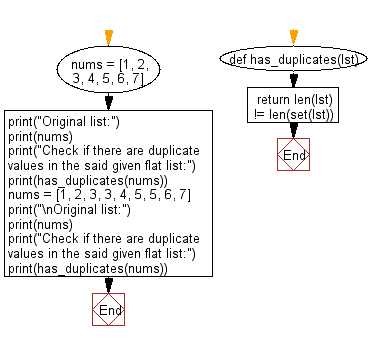﻿ Python: Check if there are duplicate values in a given flat list - w3resource# Python: Check if there are duplicate values in a given flat list

## Python List: Exercise - 271 with Solution

Write a Python program to check if there are duplicate values in a given flat list.

Use set() on the given list to remove duplicates, compare its length with the length of the list.

Sample Solution:

Python Code:

``````def has_duplicates(lst):
return len(lst) != len(set(lst))
nums = [1, 2, 3, 4, 5, 6, 7]
print("Original list:")
print(nums)
print("Check if there are duplicate values in the said given flat list:")
print(has_duplicates(nums))
nums = [1, 2, 3, 3, 4, 5, 5, 6, 7]
print("\nOriginal list:")
print(nums)
print("Check if there are duplicate values in the said given flat list:")
print(has_duplicates(nums))
```
```

Sample Output:

```Original list:
[1, 2, 3, 4, 5, 6, 7]
Check if there are duplicate values in the said given flat list:
False

Original list:
[1, 2, 3, 3, 4, 5, 5, 6, 7]
Check if there are duplicate values in the said given flat list:
True
```

Flowchart:## Visualize Python code execution:

The following tool visualize what the computer is doing step-by-step as it executes the said program:

Python Code Editor:

Have another way to solve this solution? Contribute your code (and comments) through Disqus.

What is the difficulty level of this exercise?

Test your Python skills with w3resource's quiz

﻿

## Python: Tips of the Day

Floor Division:

When we speak of division we normally mean (/) float division operator, this will give a precise result in float format with decimals.

For a rounded integer result there is (//) floor division operator in Python. Floor division will only give integer results that are round numbers.

```print(1000 // 300)
print(1000 / 300)```

Output:

```3
3.3333333333333335```